# How do I read my meter?

We rely on you submitting regular meter readings to ensure your bills are accurate. First things first Find your meter.

Next up is to work out what type of meter you have the simplest way to do this is to look at the following; The number of dials, the type of dial, or the type of display.

then follow the simple steps below:

# Gas Meters

Metric Meter

Simply read the first five numbers from left to right. Include any 0's at the beginning, but ignore any red numbers or numbers after the decimal point or space.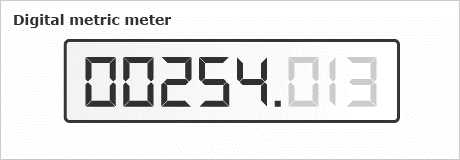In the example above the Meter reading is 00254.

Imperial Meter

Read the first four numbers from left to right. Include any 0's at the beginning, but ignore any red numbers or numbers after the decimal point or space.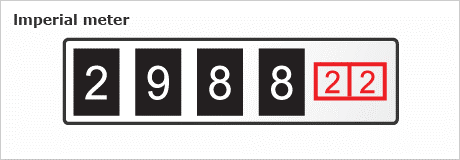In the example above the Meter reading is 2988.

Dial Meter

This is slightly trickier than newer meters; Read the four dials from left to right along the bottom row only – ignore any others and write down the number closest to each pointer. When the pointer is between two numbers, write down the lower number. If the pointer is between 9 and 0 write down 9. Remember each dial turns in the opposite direction to the one before it, so check which way each turns.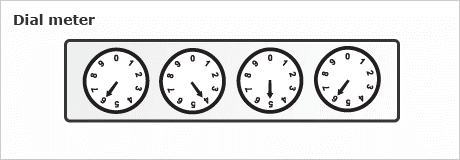In the example above the Meter reading is  6456

# Electric Meters:

Single rate meters

This meter is simply read by reading the numbers left to right, ignoring any numbers in red. this one may be obvious but other meters can be more complicated.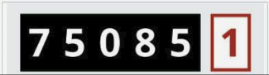In the example above the Meter reading is 75085.

## Two rate meter:

Two rate meters come in 2 different forms, Dual display which shows your rates on two separate dials or single display which will show the reads on one screen. Identify which one you have and check below to see what you need from it.

### Dual display

Both rates will be displayed either, we need both these numbers as they are your Low/Night and Normal/Day rates.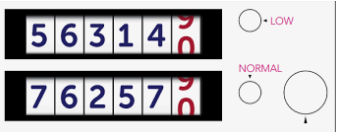### Single Display

We will need both meter readings, most single displays will cycle through automatically, some may need you to press a button.It will display 2 or 3 different values. Rate 1 (R1), rate 2 (R2) and then total (TOT,T) we only need R1 and R2.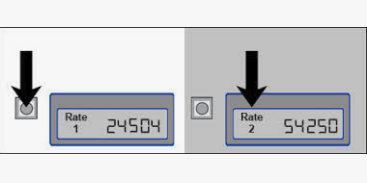In the example above the Meter reading is, rate 1 24504 and rate 2 reads 54250

## Dial meter

This is slightly trickier than newer meters; Read the Five dials from left to right  – ignore any in red or the dial 1/1.

Write down the number closest to each pointer. When the pointer is between two numbers, write down the lower number.

If the pointer is exactly on a number but the reading on the dial  after it is nine, take one away from that number.

Remember each dial turns in the opposite direction to the one before it, so check which way each turns.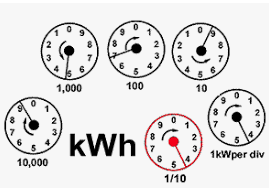In the example above the Meter reading is 95794

I Still don’t get it?

Don’t worry, take a photo using a camera or smart phone and email it to us at help@enticeenergy.com

We’ll do the rest.